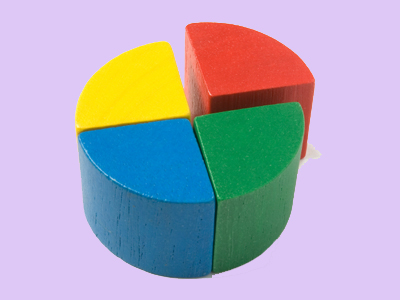If something is quartered, it is cut into 4 pieces.

# Fractions 3 (Very Easy)

Fractions – it’s a word that can strike terror in some people. But, if you’ve already played our previous quizzes on the topic, this one will not be too difficult. It will test your ability to add, subtract and divide fractions, and will also see how good you are at converting one fraction into another.

Basically, fractions are made of two numbers: the denominator (the bottom number) tells you how many ‘pieces’ a whole has been cut into, and the numerator (the top number) tells you how many of those pieces the fraction represents. For example, in 14, 4 is the denominator (a whole is cut into four pieces) and 1 is the numerator (a quarter represents one of those pieces).

Okay then, now we’ve honed our wits, it’s time for the quiz. Good luck!

1.
What does 921 + 521 equal?
34
13
12
23
If the denominators (bottom numbers) are the same, you can add by simply adding their numerators (top numbers). 921 + 521 = 1421 BUT this can be reduced to 23 if you divide the denominator and the numerator by the SAME number, in this case 7
2.
Which one of the fractions below is the smallest?
936
618
1030
26
The three wrong answers can be reduced to 13 which is greater than 936 or 14
3.
Which one of the fractions below is the biggest?
212
318
424
728
All the other fractions can be reduced to 16 which is smaller than 728 or 14
4.
Which one of the following fractions is NOT the same as 15
210
640
420
840
640 could be simplified to 320 which is smaller than 420 which is the same as 15
5.
Which one of the following fractions is the same as nine-twelfths (912)?
23
56
34
12
If you divide the denominator and the numerator by the SAME number, you can reduce the fraction to its simplest form. In this case, you divide the denominator AND the numerator by 3
6.
In an election, 30,000 people voted. If one-quarter of them voted for the Blue Party, how many did not vote for the Blue Party?
22,500
20,000
7,500
10,000
14 of 30,000 voted for the Blue Party. So, 30,000 ÷ 4 = 7,500. That means 30,000 - 7,500 voted for other parties. 30,000 - 7,500 = 22,500
7.
What does 1511711 equal?
23
811
34
411
If the denominators (bottom numbers) are the same, you can subtract by simply subtracting their numerators (top numbers). 1511711 = 811
8.
If 1216 of the class like football. What fraction of the class do not like football?
13
816
14
412
If 1216 of the class like football, then 416 of the class do not like football because 12 + 4 = 16: this can be simplified to 14 by dividing by 4
9.
If you eat 29 of a pizza, how much pizza will be left?
13
14
79
718
There are nine ninths in 1. If you have eaten 29, there can only be 79 left because 2 + 7 = 9: if the denominators are the same, you can add/subtract the fractions by simply adding/subtracting their numerators
10.
What is the value of this fraction: 628
Six twenty-eighths
Sixth twenty-eighths
Six twenty-eights
Six twenty-eights
Numbers followed by th, st or rd (as in, fourth, first or third) are known as ordinal numbers. The denominators (bottom numbers) in fractions are almost always ordinal numbers
Author:  Frank Evans

We use cookies to make your experience of our website better.

To comply with the new e-Privacy directive, we need to ask for your consent -